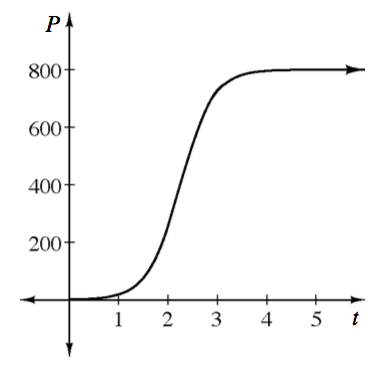### Home > APCALC > Chapter 12 > Lesson 12.1.2 > Problem12-24

12-24.

Multiple Choice: The graph at right shows the growth of a population $P$ of sea lions introduced near a remote island after $t$ years. A reasonable differential equation to model this growth is:

1. $\frac { d P } { d t } = \frac { 1 } { 800 } P ( 400 - P )$

1. $\frac { d P } { d t } = \frac { 1 } { 400 } P ( 800 - P )$

1. $\frac { d P } { d t } = \frac { 1 } { 800 } P$

1. $\frac { d P } { d t } = \frac { 1 } { 400 } ( 800 - P )$

1. $\frac { d P } { d t } = \frac { 1 } { 800 } ( 400 - P )$$\frac{dP}{dt} = 0\text{ when }P = 0\text{ and }800.$

Which equation satisfies these conditions?